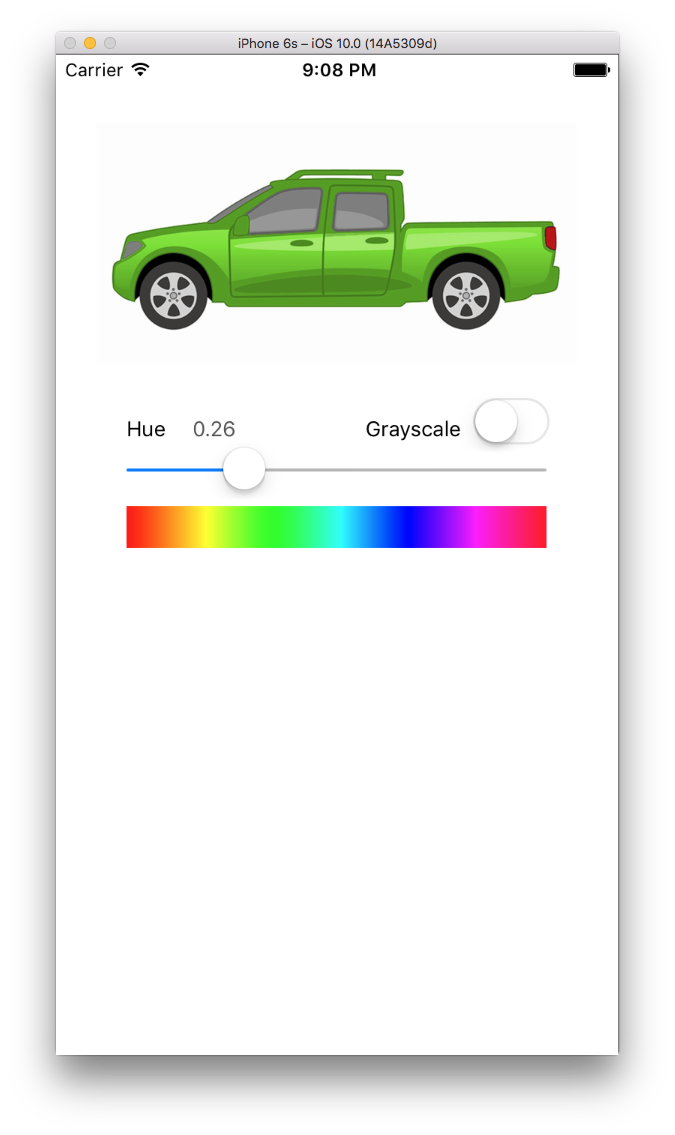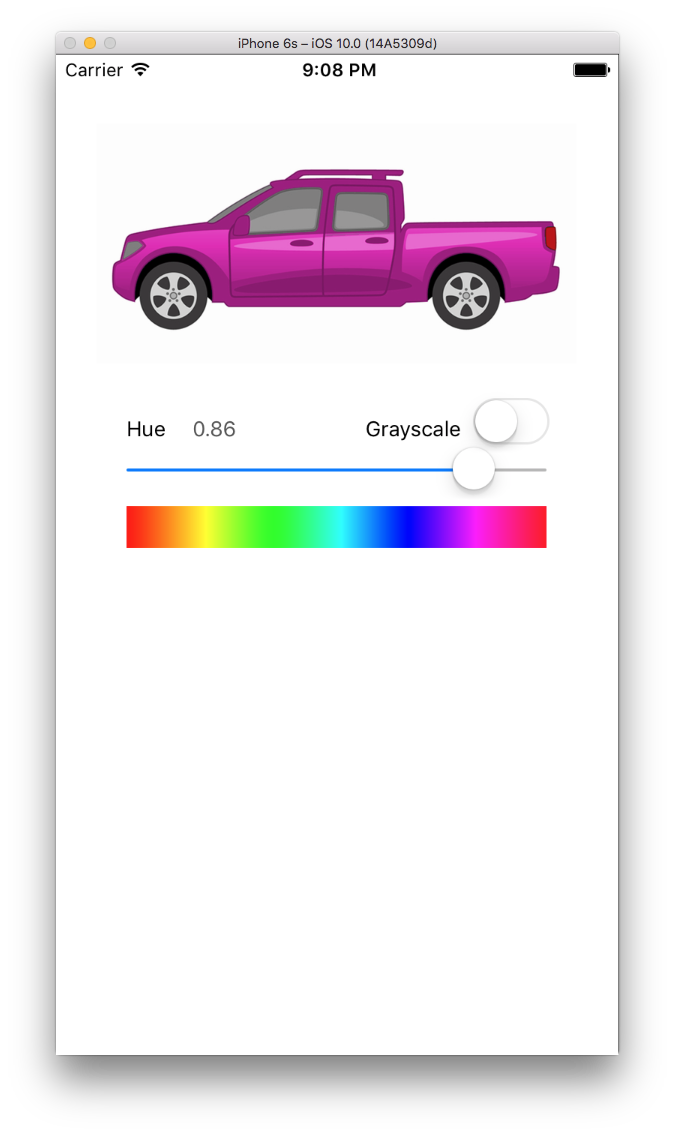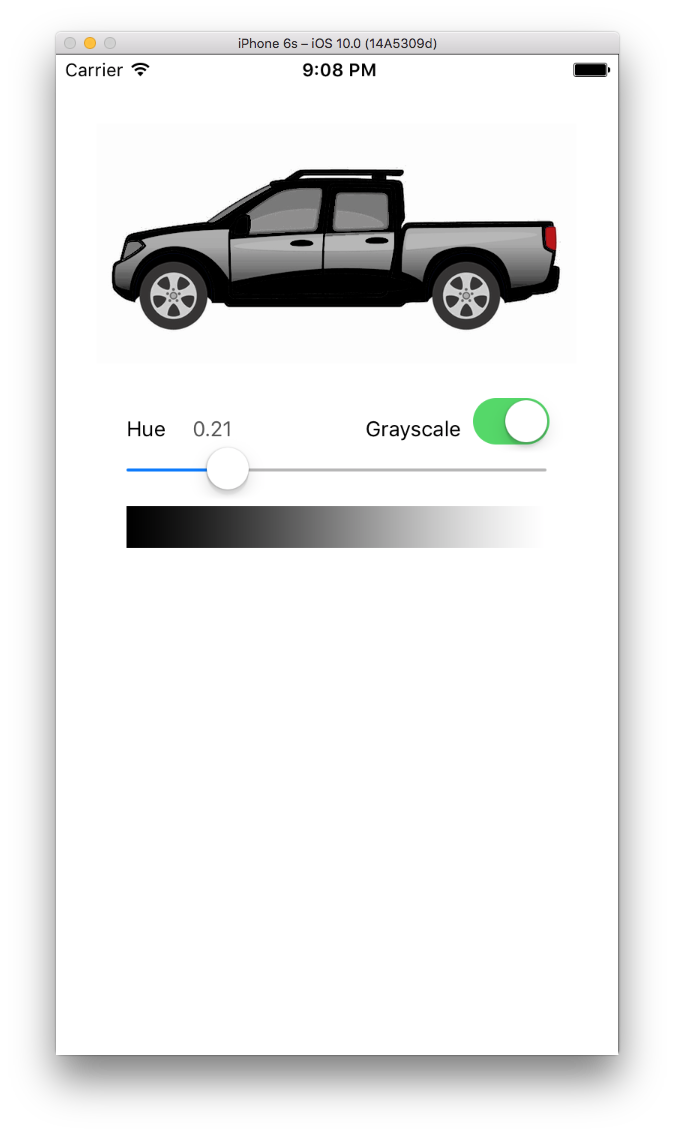# How to change a particular color in an image?

My question is if I have a Lion image just I want to change the color of the lion alone not the background color. For that I referred this SO question but it turns the color of whole image. Moreover the image is not looking great. I need the color change like photoshop. whether it is possible to do this in coregraphics or I have to use any other library.

EDIT : I need the color change to be like iQuikColor app• nice question, if u got the perfect answer, i'll use that – vijay gupta Nov 8 '11 at 6:30
• i'm also having the same issue, i posted the question colorfill issue, – Marios Nov 15 '11 at 6:48
• hi did you got any idea in this – Marios Nov 15 '11 at 15:40
• I am trying jano's answer.. now got struck in 3 rd point... – Aravindhan Nov 15 '11 at 16:35
• @Rocotilos can you help please? I am trying for the same.. – slaveCoder Dec 18 '13 at 9:11

Here is the sketch of a possible solution using OpenCV:

• Convert the image from RGB to HSV using `cvCvtColor` (we only want to change the hue).
• Isolate a color with `cvThreshold` specifying a certain tolerance (you want a range of colors, not one flat color).
• Discard areas of color below a minimum size using a blob detection library like cvBlobsLib. This will get rid of dots of the similar color in the scene.
• Mask the color with `cvInRangeS` and use the resulting mask to apply the new hue.
• `cvMerge` the new image with the new hue with an image composed by the saturation and brightness channels that you saved in step one.

There are several OpenCV iOS ports in the net, eg: http://www.eosgarden.com/en/opensource/opencv-ios/overview/ I haven't tried this myself, but seems a good research direction.

• Hi jano, I am stuck in step:4, I have applied cvInRangeS function and I got threashold image but not sure what do you mean by "use the resulting mask to apply the new hue." and how to use in step:5? can you please elaborate more about it – Iducool Feb 26 '13 at 10:30

This took quite a while to figure out, mainly because I wanted to get it up and running in Swift using Core Image and CIColorCube.

@Miguel's explanation is spot on about the way you need to replace a "Hue angle range" with another "Hue angle range". You can read his post above for details on what a Hue Angle Range is.

I made a quick app that replaces a default blue truck below, with whatever you choose on Hue slider.You can slide the slider to tell the app what color Hue you want to replace the blue with.

I'm hardcoding the Hue range to be 60 degrees, which typically seems to encompass most of a particular color but you can edit that if you need to.Notice that it does not color the tires or the tail lights because that's outside of the 60 degree range of the truck's default blue hue, but it does handle shading appropriately.

First you need code to convert RGB to HSV (Hue value):

``````func RGBtoHSV(r : Float, g : Float, b : Float) -> (h : Float, s : Float, v : Float) {
var h : CGFloat = 0
var s : CGFloat = 0
var v : CGFloat = 0
let col = UIColor(red: CGFloat(r), green: CGFloat(g), blue: CGFloat(b), alpha: 1.0)
col.getHue(&h, saturation: &s, brightness: &v, alpha: nil)
return (Float(h), Float(s), Float(v))
}
``````

Then you need to convert HSV to RGB. You want to use this when you discover a hue that in your desired hue range (aka, a color that's the same blue hue of the default truck) to save off any adjustments you make.

``````func HSVtoRGB(h : Float, s : Float, v : Float) -> (r : Float, g : Float, b : Float) {
var r : Float = 0
var g : Float = 0
var b : Float = 0
let C = s * v
let HS = h * 6.0
let X = C * (1.0 - fabsf(fmodf(HS, 2.0) - 1.0))
if (HS >= 0 && HS < 1) {
r = C
g = X
b = 0
} else if (HS >= 1 && HS < 2) {
r = X
g = C
b = 0
} else if (HS >= 2 && HS < 3) {
r = 0
g = C
b = X
} else if (HS >= 3 && HS < 4) {
r = 0
g = X
b = C
} else if (HS >= 4 && HS < 5) {
r = X
g = 0
b = C
} else if (HS >= 5 && HS < 6) {
r = C
g = 0
b = X
}
let m = v - C
r += m
g += m
b += m
return (r, g, b)
}
``````

Now you simply loop through a full RGBA color cube and "adjust" any colors in the "default blue" hue range with those from your newly desired hue. Then use Core Image and the CIColorCube filter to apply your adjusted color cube to the image.

``````func render() {
let centerHueAngle: Float = 214.0/360.0 //default color of truck body blue
let destCenterHueAngle: Float = slider.value
let minHueAngle: Float = (214.0 - 60.0/2.0) / 360 //60 degree range = +30 -30
let maxHueAngle: Float = (214.0 + 60.0/2.0) / 360
var hueAdjustment = centerHueAngle - destCenterHueAngle
let size = 64
var cubeData = [Float](count: size * size * size * 4, repeatedValue: 0)
var rgb: [Float] = [0, 0, 0]
var hsv: (h : Float, s : Float, v : Float)
var newRGB: (r : Float, g : Float, b : Float)
var offset = 0
for var z = 0; z < size; z++ {
rgb = Float(z) / Float(size) // blue value
for var y = 0; y < size; y++ {
rgb = Float(y) / Float(size) // green value
for var x = 0; x < size; x++ {
rgb = Float(x) / Float(size) // red value
hsv = RGBtoHSV(rgb, g: rgb, b: rgb)
if hsv.h < minHueAngle || hsv.h > maxHueAngle {
newRGB.r = rgb
newRGB.g = rgb
newRGB.b = rgb
} else {
hsv.h = destCenterHueAngle == 1 ? 0 : hsv.h - hueAdjustment //force red if slider angle is 360
newRGB = HSVtoRGB(hsv.h, s:hsv.s, v:hsv.v)
}
cubeData[offset]   = newRGB.r
cubeData[offset+1] = newRGB.g
cubeData[offset+2] = newRGB.b
cubeData[offset+3] = 1.0
offset += 4
}
}
}
let data = NSData(bytes: cubeData, length: cubeData.count * sizeof(Float))
let colorCube = CIFilter(name: "CIColorCube")!
colorCube.setValue(size, forKey: "inputCubeDimension")
colorCube.setValue(data, forKey: "inputCubeData")
colorCube.setValue(ciImage, forKey: kCIInputImageKey)
if let outImage = colorCube.outputImage {
let context = CIContext(options: nil)
let outputImageRef = context.createCGImage(outImage, fromRect: outImage.extent)
imageView.image = UIImage(CGImage: outputImageRef)
}
}
``````

• Outstanding post! (voted.) Now I just need to find the time to download the project and figure out how your converting your HSV color ranges into a color cube. Why not make the input hue a slider as well, and also the hue angle range? That would make for a more flexible sample, and shouldn't be that hard to do. – Duncan C Sep 17 '15 at 22:04
• I wrote a project a while back (pre-swift) that interrogates iOS for a list of available CI filters and builds a UI to collect the inputs to try most of them out. I didn't spend the time to figure out how to build a color cube, so it was one of the ones my app doesn't support. Your post might give me the nudge I need to add support for the color cube filter. The results certainly look very good. – Duncan C Sep 17 '15 at 22:05
• What do you mean by a "white or gray hue"? Do you mean creating a monochrome car? You probably need to adjust the saturation value in the destination color range rather than the hue value. I'm guessing you'd still use a hue angle range for the input colors, and then knock down the saturation on the output colors. – Duncan C Sep 17 '15 at 22:11
• How did you figure out how to generate the color cube data? The Xcode docs on the CIColorCube filter leave a great deal to the imagination, to the point that I could not make any sense of them. – Duncan C Sep 18 '15 at 0:18
• Can you point me to some docs that explain how to construct a color cube? From your code it looks like each coordinate in the cube contains values ranging from 0 to 1 in R/G/B/A. Are the x,y,z coordinates of each point the source color value, and the value stored there is the output color? And then it interpolates values between? – Duncan C Sep 18 '15 at 0:25

I'm going to make the assumption that you know how to perform these basic operations, so these won't be included in my solution:

• get the RGB value of a given pixel of the loaded image
• set the RGB value of a given pixel
• display a loaded image, and/or save it back to disk.

First of all, let's consider how you can describe the source and destination colors. Clearly you can't specify these as exact RGB values, since a photo will have slight variations in color. For example, the green pixels in the truck picture you posted are not all exactly the same shade of green. The RGB color model isn't very good at expressing basic color characteristics, so you will get much better results if you convert the pixels to HSL. Here are C functions to convert RGB to HSL and back.

The HSL color model describes three aspects of a color:

1. Hue - the main perceived color - i.e. red, green, orange, etc.
2. Saturation - how "full" the color is - i.e. from full color to no color at all
3. Lightness - how bright the color is

So for example, if you wanted to find all the green pixels in a picture, you will convert each pixel from RGB to HSL, then look for H values that correspond to green, with some tolerance for "near green" colors. Below is a Hue chart, from Wikipedia:So in your case you will be looking at pixels that have a Hue of 120 degrees +/- some amount. The bigger the range the more colors will get selected. If you make your range too wide you will start seeing yellow and cyan pixels getting selected, so you'll have to find the right range, and you may even want to offer the user of your app controls to select this range.

In addition to selecting by Hue, you may want to allow ranges for Saturation and Lightness, so that you can optionally put more limits to the pixels that you want to select for colorization.

Finally, you may want to offer the user the ability to draw a "lasso selection" so that specific parts of the picture can be left out of the colorization. This is how you could tell the app that you want the body of the green truck, but not the green wheel.

Once you know which pixels you want to modify it's time to alter their color.

The easiest way to colorize the pixels is to just change the Hue, leaving the Saturation and Lightness from the original pixel. So for example, if you want to make green pixels magenta you will be adding 180 degrees to all the Hue values of the selected pixels (making sure you use modulo 360 math).

If you wanted to get more sophisticated, you can also apply changes to Saturation and that will give you a wider range of tones you can go to. I think the Lightness is better left alone, you may be able to make small adjustments and the image will still look good, but if you go too far away from the original you may start seeing hard edges where the process pixels border with background pixels.

Once you have the colorized HSL pixel you just convert it back to RGB and write it back to the image.

I hope this helps. A final comment I should make is that Hue values in code are typically recorded in the 0-255 range, but many applications show them as a color wheel with a range of 0 to 360 degrees. Keep that in mind!

Can I suggest you look into using OpenCV? It's an open source image manipulation library, and it's got an iOS port too. There are plenty of blog posts about how to use it and set it up.

It has a whole heap of functions that will help you do a good job of what you're attempting. You could do it just using CoreGraphics, but the end result isn't going to look nearly as good as OpenCV would.

It was developed by some folks at MIT, so as you might expect it does a pretty good job at things like edge detection and object tracking. I remember reading a blog about how to separate a certain color from a picture with OpenCV - the examples showed a pretty good result. See here for an example. From there I can't imagine it would be a massive job to actually change the separated color to something else.

I don't know of a CoreGraphics operation for this, and I don't see a suitable CoreImage filter for this. If that's correct, then here's a push in the right direction:

Assuming you have a `CGImage` (or a `uiImage.CGImage`):

• Begin by creating a new `CGBitmapContext`
• Draw the source image to the bitmap context
• Get a handle to the bitmap's pixel data

Learn how the buffer is structured so you could properly populate a 2D array of pixel values which have the form:

``````typedef struct t_pixel {
uint8_t r, g, b, a;
} t_pixel;
``````

Then create the color to locate:

``````const t_pixel ColorToLocate = { 0,0,0,255 }; // << black, opaque
``````

And its substitution value:

``````const t_pixel SubstitutionColor = { 255,255,255,255 }; // << white, opaque
``````
• Iterate over the bitmap context's pixel buffer, creating `t_pixel`s.
• When you find a pixel which matches `ColorToLocate`, replace the source values with the values in `SubstitutionColor`.

• Create a new `CGImage` from the `CGBitmapContext`.

That's the easy part! All that does is takes a `CGImage`, replace exact color matches, and produces a new `CGImage`.

What you want is more sophisticated. For this task, you will want a good edge detection algorithm.

I've not used this app you have linked. If it's limited to a few colors, then they may simply be swapping channel values, paired with edge detection (keep in mind that buffers may also be represented in multiple color models - not just RGBA).

If (in the app you linked) the user can choose an arbitrary colors, values, and edge thresholds, then you will have to use real blending and edge detection. If you need to see how this is accomplished, you may want to check out a package such as Gimp (it's an open image editor) - they have the algos to detect edges and choose by color.

• I too tried like this only.. but it takes time..May be I have to use an efficient algorithm. – Aravindhan Nov 11 '11 at 4:28
• @Trisha I'd imagine a good implementation would take time for a full size image. But yes, the edge detection will likely take some time to calculate. On modern hardware, you could reasonably divide the processing among one or two secondary threads. As you say, the algo's also quite important. – justin Nov 11 '11 at 15:09